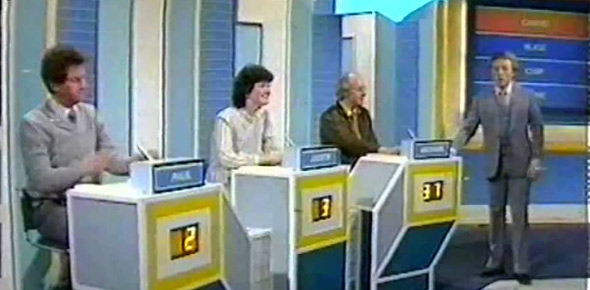# The Odd One Out Quiz!

Approved & Edited by ProProfs Editorial Team
At ProProfs Quizzes, our dedicated in-house team of experts takes pride in their work. With a sharp eye for detail, they meticulously review each quiz. This ensures that every quiz, taken by over 100 million users, meets our standards of accuracy, clarity, and engagement.
| Written by Diploman
D
Diploman
Community Contributor
Quizzes Created: 1 | Total Attempts: 203
Questions: 10 | Attempts: 204SettingsThis quiz is a quiz about Pokemon where you need to find the odd one out.

• 1.

### Which Pokemon is the odd one out? = A = B = C = D

• A.

A

• B.

B

• C.

C

• D.

D

D. D
Explanation
I bet I got you with that one! Lucario is the odd one out because it doesn't have a base 100 speed!

Rate this question:

• 2.

### Which move is the odd one out?Hydro Pump = AFire Blast = BFlamethrower = CThunder = DBlizzard = E

• A.

A

• B.

B

• C.

C

• D.

D

• E.

E

C. C
Explanation
Flamethrower is the only one without a base power of 120.

Rate this question:

• 3.

### Which move is the odd one out?Megahorn = ATriple Kick = BMeteor Mash = CBounce = DPin Missile = E

• A.

A

• B.

B

• C.

C

• D.

D

• E.

E

B. B
Explanation
Triple Kick is the only one without an 85% accuracy.

Rate this question:

• 4.

### Which type is the odd one out?Fire = ARock = BPsychic = CElectric = DDragon = E

• A.

A

• B.

B

• C.

C

• D.

D

• E.

E

B. B
Explanation
Rock wasn't a special type in RSE.

Rate this question:

• 5.

### Which effect is the odd one out?Rain = ASun = BTrick Room = CGravity = DTailwind = E

• A.

A

• B.

B

• C.

C

• D.

D

• E.

E

E. E
Explanation
Tailwind is the only one that doesn't last at least five turns, and it only effects one side of the field.

Rate this question:

• 6.

### Which ability is the odd one out?Limber = AImmunity = BOblivious = COwn Tempo = DDamp = E

• A.

A

• B.

B

• C.

C

• D.

D

• E.

E

E. E
Explanation
Damp is the only one that doesn't cause an immunity to a status.

Rate this question:

• 7.

### Which type combination is the odd one out?Rock/Dark = AWater/Ground = BFire/Flying = CDragon/Ground = DIce/Flying = E

• A.

A

• B.

B

• C.

C

• D.

D

• E.

E

A. A
Explanation
Rock/Dark because it is unique to only one Pokemon, Tyranitar. Swampert, Whiscash, Gastrodon, and Quagsire share Water/Ground. Charizard, Ho-oh, and Moltres share Fire/Flying. Garchomp and Flygon share Dragon/Ground, while Delibird and Articuno share Ice/Flying. Also note that all 5 of these type combinations have 4X weaknesses.

Rate this question:

• 8.

### Which spread of base stats is the odd one out?100/50/75/80/95/70 = A110/30/45/40/105/140 = B60/130/10/130/10/130 = C130/5/5/130/130/70 = D145/60/45/60/45/60 = E

• A.

A

• B.

B

• C.

C

• D.

D

• E.

E

E. E
Explanation
145/60/45/60/45/60 because it's the only one which doesn't have a Base Stat Total of 470. It has 475 as its Base Stat Total.

Rate this question:

• 9.

### Which Pokemon is the odd one out? = A = B = C = D = E

• A.

A

• B.

B

• C.

C

• D.

D

• E.

E

D. D
Explanation
Tropius is the answer because it is the only one without a unique type combination. Grass/Flying is a type it and Shaymin-S both have.

Rate this question:

• 10.

### Last Question! Be prepared...nah, not really.Which Pokemon Game is the odd one out?Diamond = APearl = BPlatinum = CEmerald = DRuby = E

• A.

A

• B.

B

• C.

C

• D.

D

• E.

EBack to top